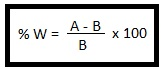# What is the Moisture Content?

Moisture Content of a sample is a percentage weight of the water content in the weight of the sample.

The moisture content of the sample is calculated using the following equation:Where:

%W = Percentage of moisture in the sample,

A = Weight of wet sample (grams), and

B = Weight of dry sample (grams)